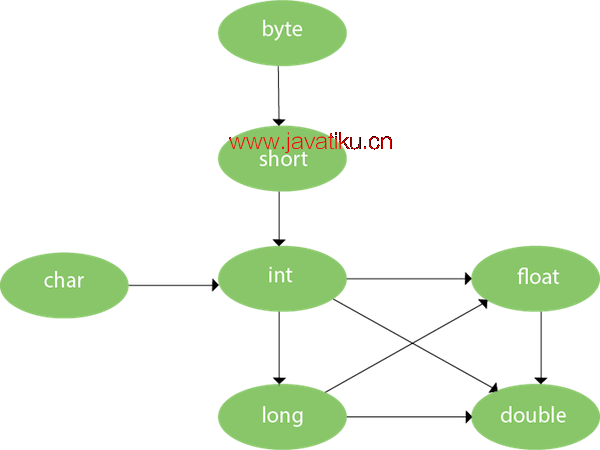# Java教程-详解Java 重载1. 通过改变参数的数量
2. 通过改变参数的数据类型

### 1）方法重载：改变参数的数量

``````class Adder{
static int add(int a,int b){return a+b;}
static int add(int a,int b,int c){return a+b+c;}
}
public static void main(String[] args){
}}  ``````

``````22
33``````

### 2）方法重载：改变参数的数据类型

``````class Adder{
static int add(int a, int b){return a+b;}
static double add(double a, double b){return a+b;}
}
public static void main(String[] args){
}}  ``````

``````22
24.9``````

### Q) 为什么仅通过改变方法的返回类型是不可能实现方法重载的？

``````class Adder{
static int add(int a,int b){return a+b;}
static double add(int a,int b){return a+b;}
}
public static void main(String[] args){
}}  ``````

``Compile Time Error: method add(int,int) is already defined in class Adder``

### 我们可以重载 java main() 方法吗？

``````class TestOverloading4{
public static void main(String[] args){System.out.println("main with String[]");}
public static void main(String args){System.out.println("main with String");}
public static void main(){System.out.println("main without args");}
}  ``````

``main with String[]``

## 方法重载和类型提升### 使用 TypePromotion 的方法重载示例

``````class OverloadingCalculation1{
void sum(int a,long b){System.out.println(a+b);}
void sum(int a,int b,int c){System.out.println(a+b+c);}

public static void main(String args[]){
obj.sum(20,20);//现在第二个 int 文字将被提升为 long
obj.sum(20,20,20);

}
}  ``````

``````40
60``````

### 如果找到匹配，使用类型提升的方法重载示例

``````class OverloadingCalculation2{
void sum(int a,int b){System.out.println("调用 int arg 方法");}
void sum(long a,long b){System.out.println("long arg method invoked");}

public static void main(String args[]){
obj.sum(20,20);//现在调用 int arg sum() 方法
}
}  ``````

``调用 int arg 方法``

### 在有歧义的情况下使用类型提升方法重载的示例

``````class OverloadingCalculation3{
void sum(int a,long b){System.out.println("a 方法被调用");}
void sum(long a,int b){System.out.println("b 方法被调用");}

public static void main(String args[]){
``Output:Compile Time Error``